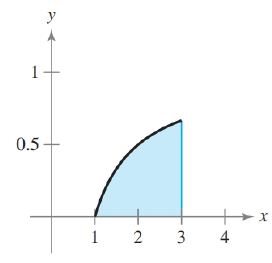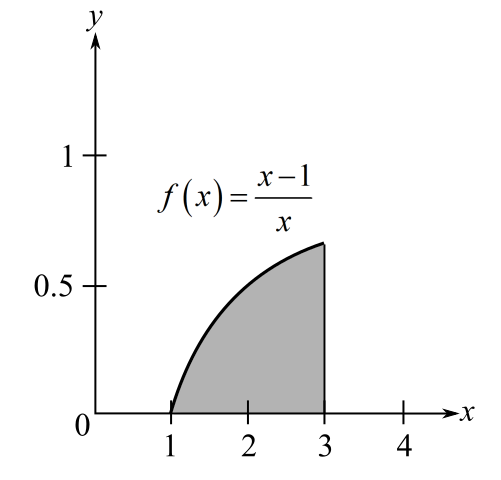Chapter 5, Problem 58RE### Calculus: An Applied Approach (Min...

10th Edition
Ron Larson
ISBN: 9781305860919

#### Solutions

Chapter
Section### Calculus: An Applied Approach (Min...

10th Edition
Ron Larson
ISBN: 9781305860919
Textbook Problem
1 views

# Finding Area by the Fundamental Theorem In Exercises 53-58, find the area of the region. f ( x ) = x − 1 xTo determine

To calculate: The area of region bounded by graph of function f(x)=x1xExplanation

Given Information:

The provided graph of function f(x)=x1x.

Formula used:

Area of function f(x) bound from x=a and x=b is given by

Area=abf(x)dx

The fundamental theorem of calculus:

abf(x)dx=F(b)F(a)

Here, F is function such that F(x)=f(x) for all x in [a,b].

Calculation:

The provided graph of function f(x)=x1x.

The graph of function f(x)=x1x is hyperbola bounded from x=1 and x=3. The integration of function f(x)=x1x from x=1 and x=3 represent the area of region bound graph

### Still sussing out bartleby?

Check out a sample textbook solution.

See a sample solution

#### The Solution to Your Study Problems

Bartleby provides explanations to thousands of textbook problems written by our experts, many with advanced degrees!

Get Started

#### If y=xa212a21arctansinxa+a21+cosx show that y=1a+cosx

Single Variable Calculus: Early Transcendentals, Volume I

#### Solve the equations in problem 1-6. 2.

Mathematical Applications for the Management, Life, and Social Sciences

#### Calculate y'. 10. y = emx' cos nx

Single Variable Calculus: Early Transcendentals

#### limx(12)x2= a) 0 b) 1 c) d) does not exist

Study Guide for Stewart's Single Variable Calculus: Early Transcendentals, 8th

#### The third partial sum of is:

Study Guide for Stewart's Multivariable Calculus, 8th